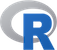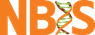@# Introduction

There are different data modes used in R. The mode of a variable will for example determine what kind of operators that can be done on it. At the end of this exercise you should know:

• What are the data types commonly used in R and how to create them
• Use some basic operators in R
• Understand how R coerce data if needed
• Basic text manipulations

## Data types

From the lectures you might remember that all elements in any data stuctures found in R will be of a certain type (or have a certain mode). The four most commonly used data types in R are: logical, integer, double (often called numeric), and character. The names hints at what they are.

• Logical = TRUE or FALSE (or NA)
• Integer = Numbers that can be represented without fractional component
• Numeric = Any number that is not a complex number.
• Character = Text

In many cases the mode of on entry is determined by the content so if you save the value 5.1 as a variable in R, the variable will automatically be recognised as numeric. If you instead have a text string like “hello world” it will have the mode character. Below you will also see examples of how you can specify the mode and not rely on R inferring the right mode based on content.

## Basic R operators

As in other programming languages there are a set of basic operators in R. Some of these are not used until later in the course, but is included here as some kind of reference information.

Operation Description Example Example Result
x + y Addition 1 + 3 4
x - y Subtraction 1 - 3 -2
x * y Multiplication 2 * 3 6
x / y Division 1 / 2 0.5
x ^ y Exponent 2 ^ 2 4
x %% y Modular arethmetic 1 %% 2 1
x %/% y Integer division 1 %/% 2 0
x == y Test for equality 1 == 1 TRUE
x <= y Test less or equal 1 <= 1 TRUE
x >= y Test for greater or equal 1 >= 2 FALSE
x && y Non-vectorized boolean AND 3 >= 2 && 3 < 10 TRUE
x & y Vectorized boolean AND
x || y Non-vectorized boolean OR 1 >= 2 || 3 < 10 TRUE
x | y Vectorized boolean OR
!x Boolean not 1 != 2 TRUE

# Exercise: Basic operations and data types in R

In all exercises on this course it is important that you prior to running the commands in R, try to figure out what you expect the result to be. You should then verify that this will indeed be the qresult by running the command in an R session. In case there is a discrepency between your expectations and the actual output make sure you understand why before you move forward. If you can not figure out how to, or which command to run you can click the key to reveal example code including expected output. If you after peaking at the code and trying out things on your own have a hard time understanding what is going on, ask the TAs or or your someone sitting next to you who might have wrapped their head around the issue.

Also note that in many cases there multiple solutions that solve the problem equally well.

We do recommend to write all code in a R markdown document in R-studio as that will at the end of the course be your own R tutorial with comments and code solutions.

## Create and retrieve information about variables in R

Open R-studio and make sure to set your working directory. Double check that you do not have stored objects in your current session with the following command. This will list all objects that you have in your current R session.

``````ls()
``````

In case you have objects that you want to remove from the current session you can do so with the rm function. NB! This command will remove all objects available in your current session.

``````rm(list = ls())
``````

This command uses commands that we have not talked about yet. If you do not understand how it works now, you will do so after tomorrows lectures and exercises.

1. Create variables var1 and var2 and initialize them with two integers of choice.

Click to see how
```var1 <- 11
var2 <- 34
```

2. Add the two variables and save them as a new variable named var3 and print the result.

Click to see how
```var3 <- var1 + var2
var3

 45
```

3. Check the class, mode, and type for var1, var2, var3 and π (is found under the variable name pi in R)

Click to see how for var1
```mode(var1)

 "numeric"

class(var1)

 "numeric"

typeof(var1)

 "double"
```
Click to see how for π
```mode(pi)
class(pi)
typeof(pi)

 "numeric"
 "numeric"
 "double"
```

4. Create two character variables containing a text of choice.
• check mode, class, and type of the first one

Click to see how create character variables
```text1 <- "test1"
text2 <- "test2"
```
• add var1 to it and report the result

Click to see how to add variables
```text1 + var1

Error in text1 + var1 : non-numeric argument to binary operator
```

5. Cast var3 to integer, cast an integer variable to double, cast a string to a double.

Click to see how
```as.integer(var3)

 45

i <- 175
as.double(i)

 175

as.double(text1)

 NA
Warning message:
NAs introduced by coercion
```

6. Report floor and ceiling of π and round π to 3 decimal places.
Click to see how
```floor(pi)
 3

ceiling(pi)
 4

round(pi, digits = 3)
 3.142
```

7. Is floor of π an integer?
Click to see how
```is.integer(floor(pi))

 FALSE
```

8. Treat ‘3.56437’ string as number.
Click to see how
```as.numeric('3.56437')
```

9. Divide ∞ by - ∞
Click to see how
```-Inf/Inf

 NaN
```

10. Create two freely chosen complex numbers.
• Check that they are complex indeed.
• Add, multiply and divide one by another.
• Add an integer to their sum.
Click to see how
```c1 <- 23 + 4i
c2 <- -15 - 7i
is.complex(c1)
 TRUE
is.complex(c2)
 TRUE

c1 + c2
 8-3i

c1 / c2
 -1.361314+0.368613i

c1 + c2 + 7
 15-3i
```

11. Multiply a logical TRUE by a logical FALSE. Rise the logical true to the 7-th power.
Click to see how
```TRUE * FALSE
T^7
 0
 1
```

12. Create two character variables containing two verses of your favorite song.
• concatenate the two variables,
• paste the variables with ‘*’ as separator.
• find if ‘and’ occurs in the second line,
• substitute a word for another,
• extract substring starting at the 5th character and 5 characters long.
Click to see how
```line1 <- "Hello darkness my old friend"
line2 <- "I've come to talk to you again"
paste(line1, line2, sep = "")
 "Hello darkness my old friendI've come to talk to you again"

paste(line1, line2, sep = "*")
 "Hello darkness my old friend*I've come to talk to you again"

grep('and', line2)
integer(0)

sub('Hello', 'Goodbye', line1)
 "Goodbye darkness my old friend"

substr(line1, 5, 5 + 5)
 "o dark"
```

## R Environment

• get help for the t.test, table, locator and identify functions,
• check for all occurences of fisher.test in the docs,
• which package contains the plot.ecdf function. What does it do?
• find package ‘reshape’-related questions on StackOverflow,
• google how to load an XML file into R,
• install the ‘PTXQC’ package from GitHub,
• look up the ‘GenABEL’ vignette,
• see all the demos available for you and run one you like,
• run examples for the fisher.test,
• check out CRANs view for genetics,
• install a CRAN package of choice,
• install the R-Forge pckage ‘bigRR’